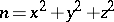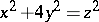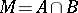# Diophantine problems of additive type

Jump to: navigation, search

Diophantine equations, for which the problem posed is to find solutions in integers, which can at the same time be considered as additive problems, i.e. as problems of decomposition of an integer(arbitrary or meeting certain additional conditions) into terms of a desired type. Such problems include, for example, solutions in integers of the following equations:(cf. Gauss number);(cf. Lagrange theorem on the sum of four squares);(cf. Integral point); as well as the Waring problem, etc. A Diophantine problem of additive type may also be regarded as the problem of finding the intersection of arithmetical sums of sets. For instance, the setof integer solutions of the equationis represented as, where